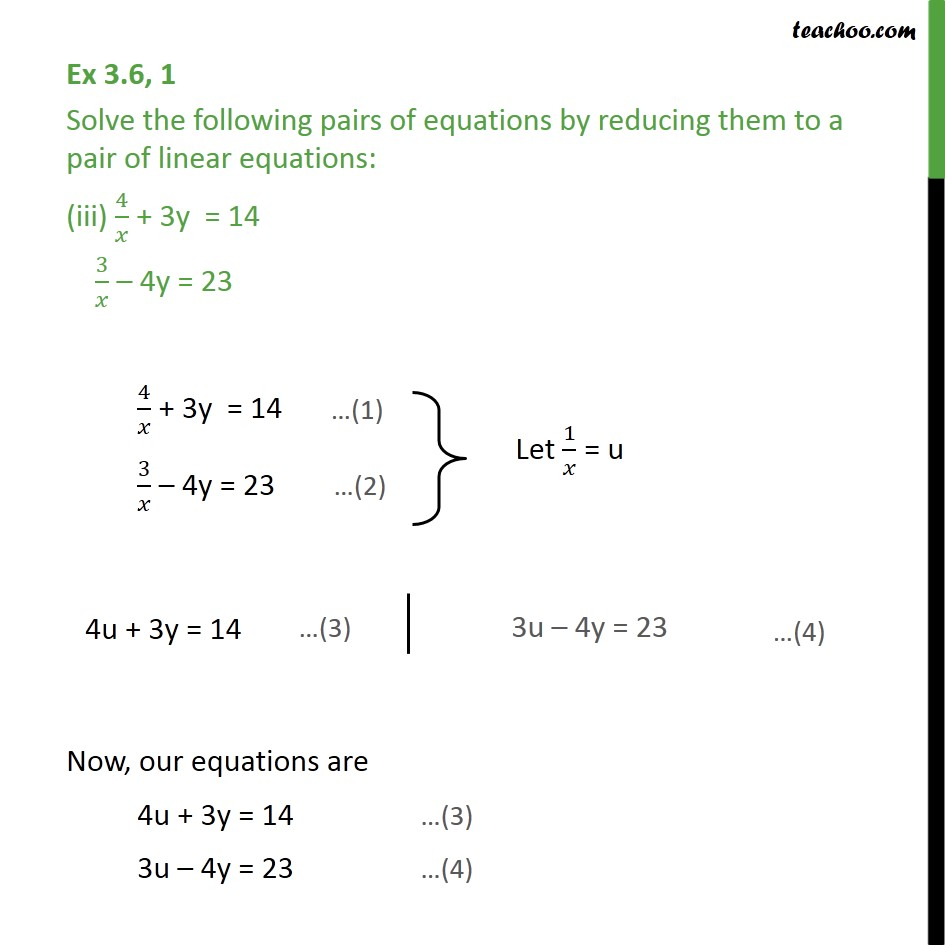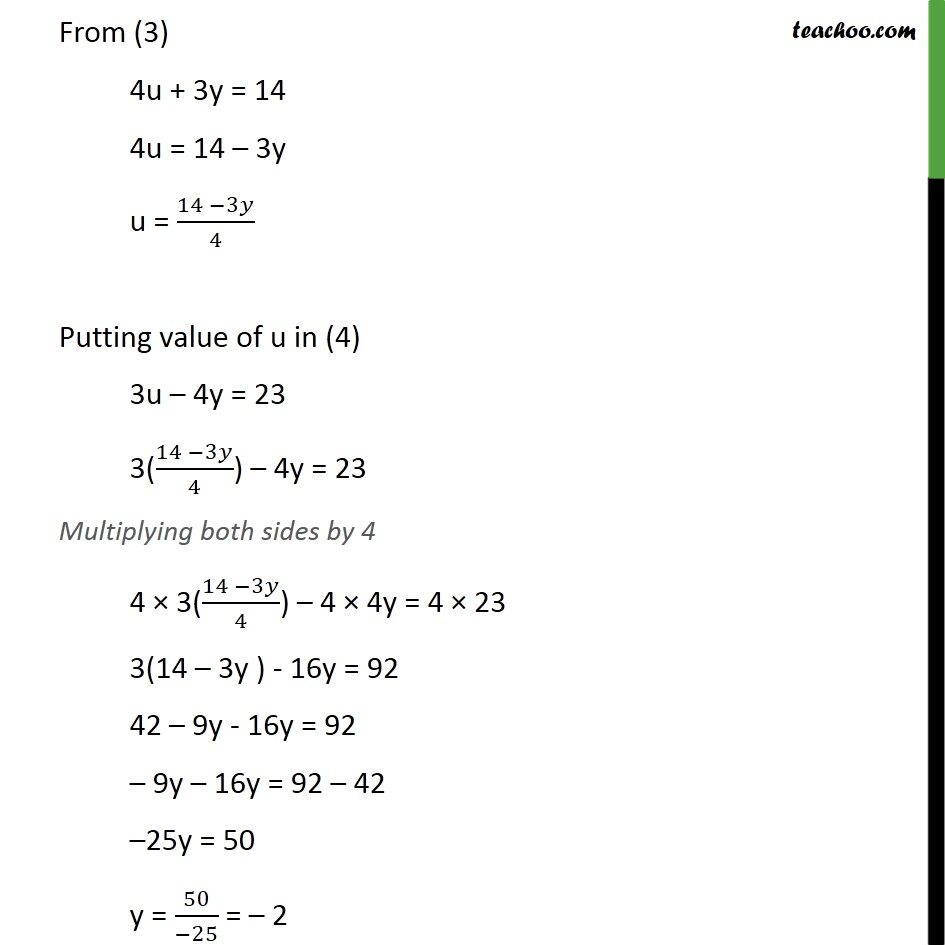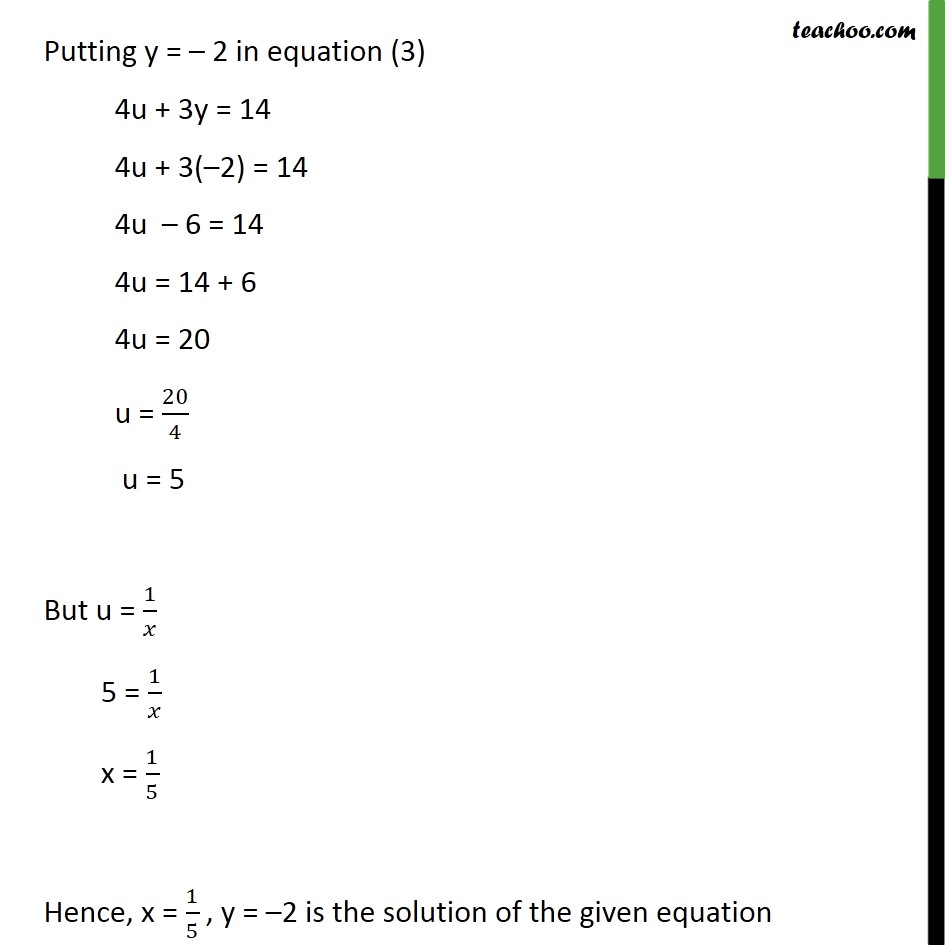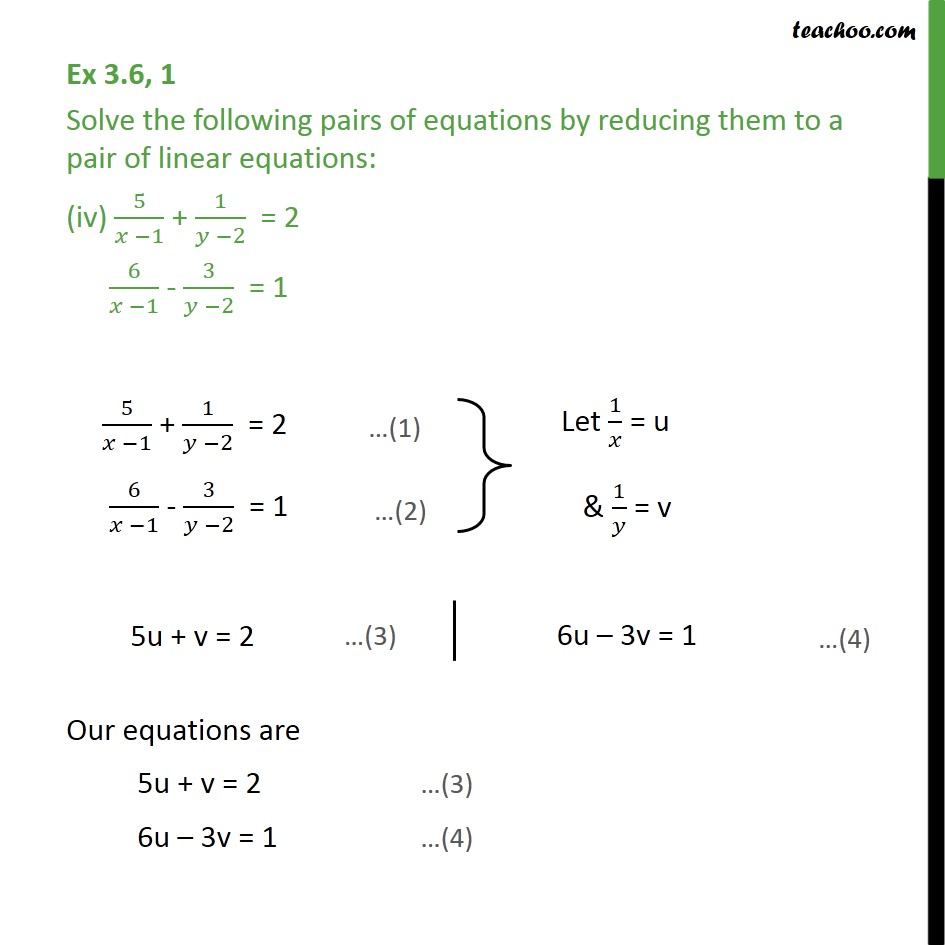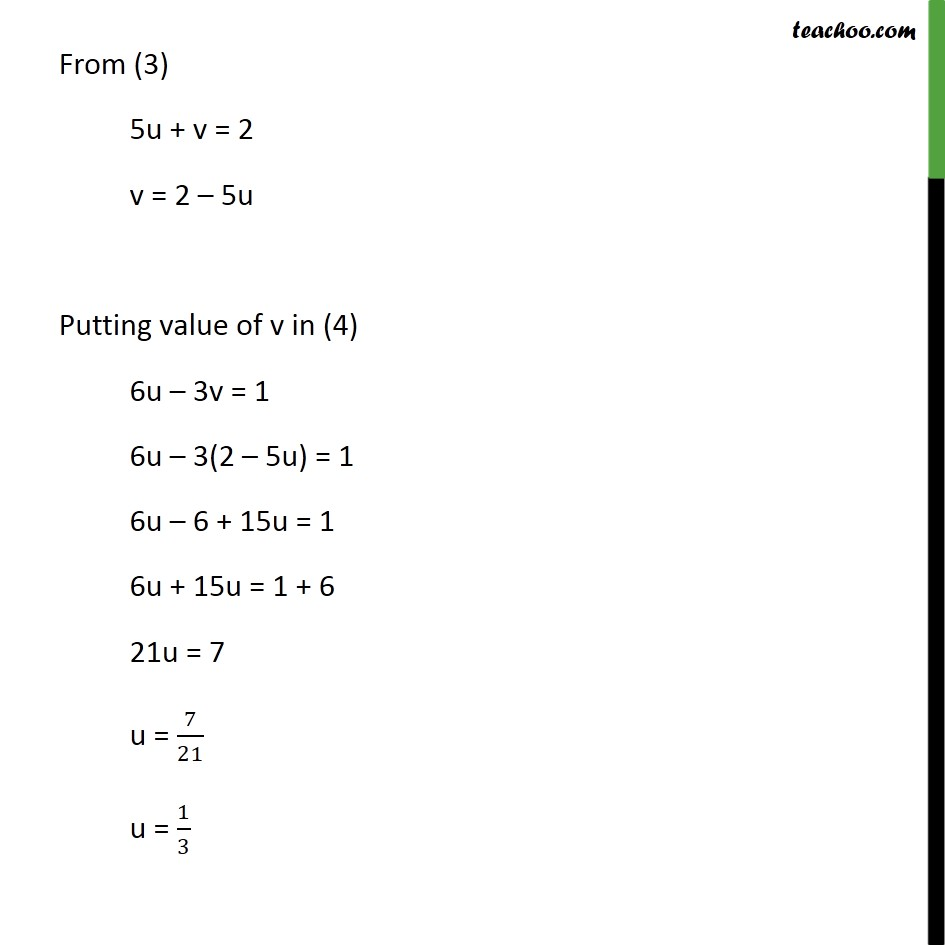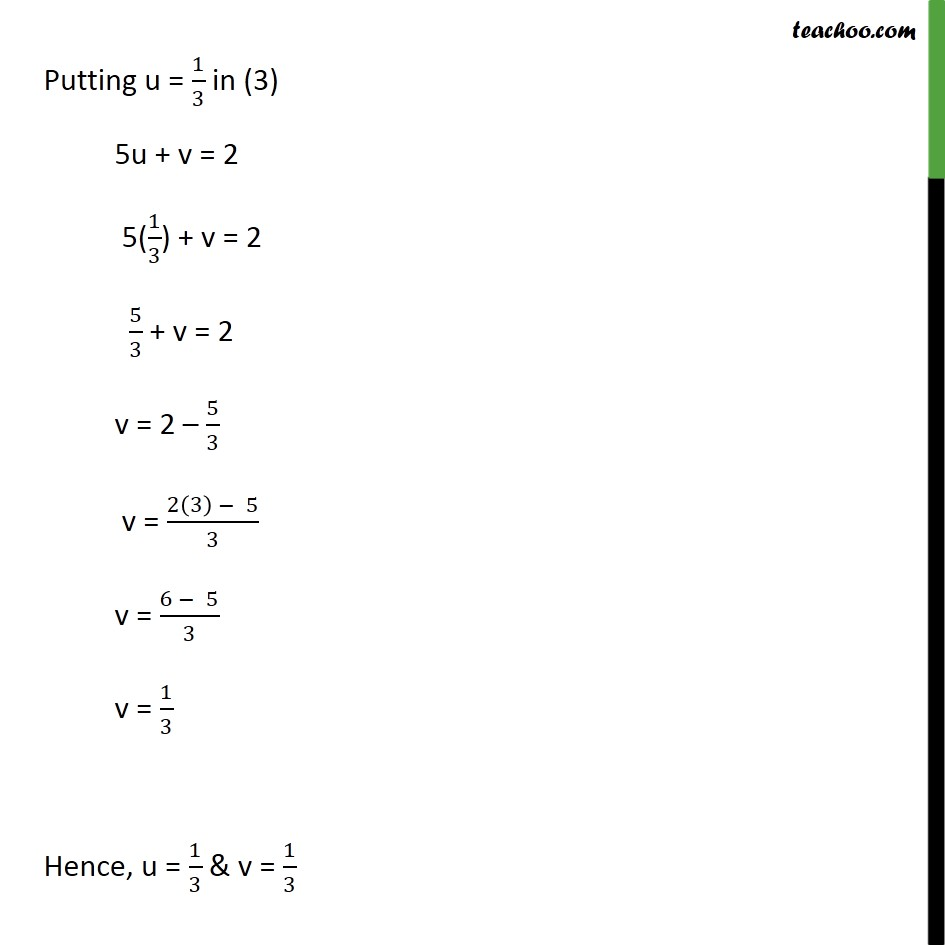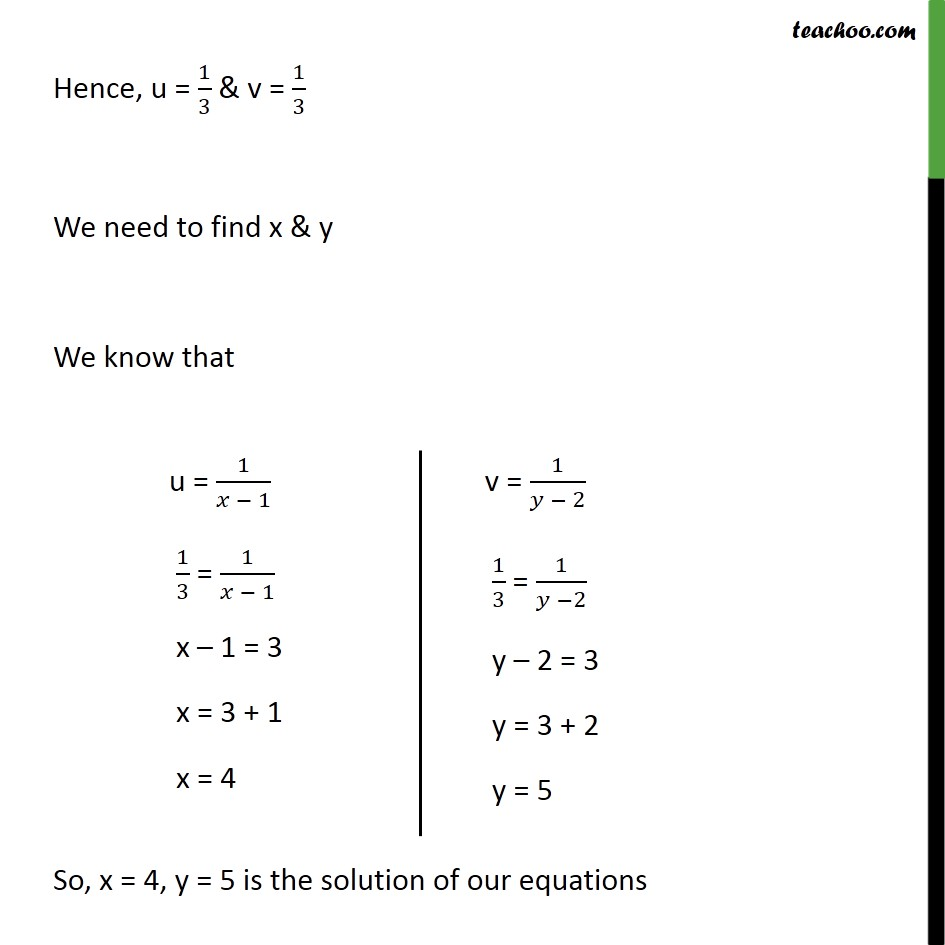1. Class 10
2. Important Questions for Exam - Class 10
3. Chapter 3 Class 10 Pair of Linear Equations in Two Variables

Transcript

Ex 3.6, 1 Solve the following pairs of equations by reducing them to a pair of linear equations: (iii) 4/𝑥 + 3y = 14 3/𝑥 – 4y = 23 4/𝑥 + 3y = 14 3/𝑥 – 4y = 23 Now, our equations are 4u + 3y = 14 …(3) 3u – 4y = 23 …(4) From (3) 4u + 3y = 14 4u = 14 – 3y u = (14 −3𝑦)/4 Putting value of u in (4) 3u – 4y = 23 3((14 −3𝑦)/4) – 4y = 23 Multiplying both sides by 4 4 × 3((14 −3𝑦)/4) – 4 × 4y = 4 × 23 3(14 – 3y ) - 16y = 92 42 – 9y - 16y = 92 – 9y – 16y = 92 – 42 –25y = 50 y = 50/(−25) = – 2 Putting y = – 2 in equation (3) 4u + 3y = 14 4u + 3(–2) = 14 4u – 6 = 14 4u = 14 + 6 4u = 20 u = 20/4 u = 5 But u = 1/𝑥 5 = 1/𝑥 x = 1/5 Hence, x = 1/5 , y = –2 is the solution of the given equation Ex 3.6, 1 Solve the following pairs of equations by reducing them to a pair of linear equations: (iv) 5/(𝑥 −1) + 1/(𝑦 −2) = 2 6/(𝑥 −1) - 3/(𝑦 −2) = 1 5/(𝑥 −1) + 1/(𝑦 −2) = 2 6/(𝑥 −1) - 3/(𝑦 −2) = 1 Our equations are 5u + v = 2 …(3) 6u – 3v = 1 …(4) From (3) 5u + v = 2 v = 2 – 5u Putting value of v in (4) 6u – 3v = 1 6u – 3(2 – 5u) = 1 6u – 6 + 15u = 1 6u + 15u = 1 + 6 21u = 7 u = 7/21 u = 1/3 Putting u = 1/3 in (3) 5u + v = 2 5(1/3) + v = 2 5/3 + v = 2 v = 2 – 5/3 v = (2(3) − 5)/3 v = (6 − 5)/3 v = 1/3 Hence, u = 1/3 & v = 1/3 Hence, u = 1/3 & v = 1/3 We need to find x & y We know that So, x = 4, y = 5 is the solution of our equations

Chapter 3 Class 10 Pair of Linear Equations in Two Variables

Class 10
Important Questions for Exam - Class 10